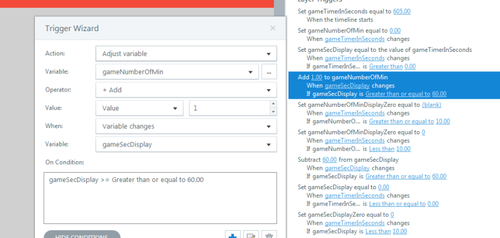# Creating a digital clock game timer display in Storyline 2

Creating an elearning game in Storyline 2 requires a lot of attention to detail to create the perfect result.  Like most things in life, breaking it down into smaller chunks can make it less demanding.

Last time, I looked at how to create a timer mechanism using two sound clips. But what if you want to display the number of seconds as a digital clock?

Using Storyline 2’s built in maths-based actions, you can transform any number into a time by following a handful of steps.

## Prerequisites

My earlier blog on Game Timer Basics in Storyline 2 outlined how to set up a timer in Storyline 2 and this post explains how to convert this single variable into a digital timer display. However, you can apply the same steps to other examples as long as you have a number to start with.

## Converting the time process

As Storyline 2 uses built in triggers rather than the type of code you would get from something like Flash or JavaScript, I’ll explain the process using pseudo-code:

• Make a count of the number of times you can subtract 60 from the total time number before it becomes less than 60. This gives us how many whole minutes we have.
• Make a note of the amount of seconds left over after doing the calculation. This gives us how many seconds we have.
• If the number of minutes or seconds is below 10 then put a zero before the number. For example, 7 becomes 07.
• Finally, combine them to display on screen with a colon to separate minutes and seconds. This simply displays the time as though it was a digital clock.

## Working Example

Let’s start with the number of seconds at 605 and run through an example:

• Count how many times you can subtract 60 from 605 until the number is less than 60 = 10
• Make a note of how many seconds are left over = 5
• Add a number before either result if its below 10 = 05
• Combine the results = 10:05It is definitely possible to apply this to Storyline 2 - I’ve done it!  If you have any problems or need further hints, please leave a comment below.

Author: Stephen Hodge, Elearning Developer at Sponge.